#There are 366 different Starters of The Day, many to choose from. You will find below some starters on the topic of Memory. A lesson starter does not have to be on the same topic as the main part of the lesson or the topic of the previous lesson. It is often very useful to revise or explore other concepts by using a starter based on a totally different area of Mathematics.

Main Page

### Memory Starters: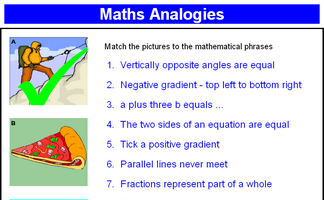Make more of analogies to help remember mathematical concepts.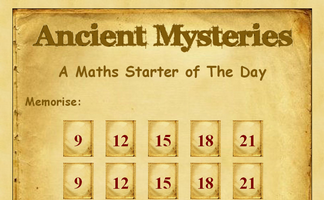This activity requires students to memorise fifteen numbers in a three by five grid.This is the Maths version of the traditional memory game. Memorise 10 mathematical facts then recall them as they disappear from view.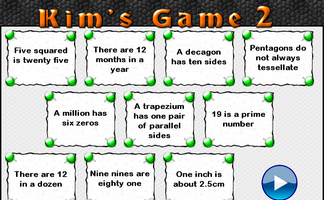This is the Maths version of the traditional memory game. Memorise 10 mathematical facts then recall them as they disappear from view.This is the Maths version of the traditional memory game. Memorise 10 mathematical facts then recall them as they disappear from view.Memorise a picture made up of geometrical shapes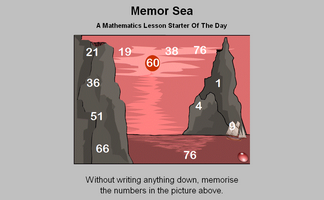Twelve numbers in a seascape need to be memorised in order to answer the five questions.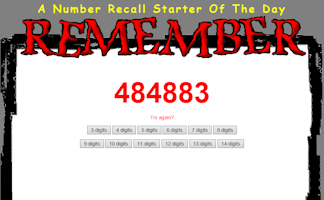Can you improve your ability to remember telephone numbers?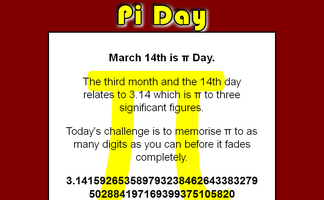March 14th is Pi Day. The third month and the 14th day relates to 3.14 which is pi to three significant figures. How many figures of pi can you memorise?A quick quiz about five items on a shopping list written 40 years ago.

## Exercises#### Algebra Pairs

The classic Pelmanism or pairs game requiring you to match equivalent expressions.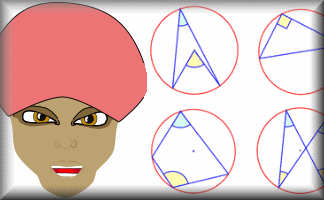#### Angle Theorem Kim's Game

A memory game to be projected to help the whole class revise the circle angle theorems.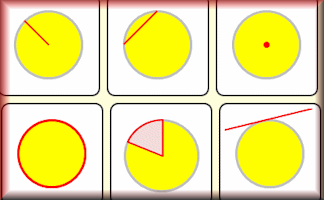#### Circle Pairs

Find the matching pairs of circle diagrams and circle properties in this interactive online game.#### Circle Parts Kim's Game

A memory game to be projected to help the whole class revise the names for the parts of a circle.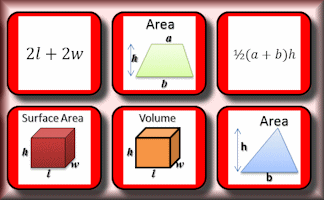#### Formulae Pairs

Find the matching pairs of diagrams and formulae for basic geometrical shapes.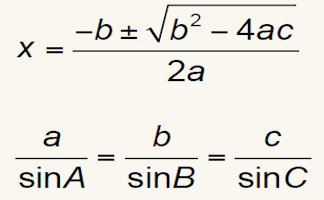#### Formulae to Remember

The traditional pairs or pelmanism game adapted to test recognition for formulae required to be memorised for GCSE exams.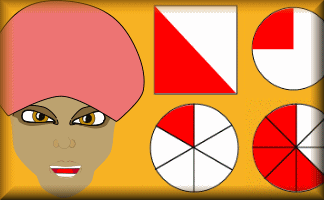#### Fractions Kim's Game

A memory game to be projected to help the whole class recognise basic fractions.#### Indices Pairs

The traditional pairs or pelmanism game adapted to test knowledge of indices.#### Kim's Quiz

The traditional memory game played with mathematical statements.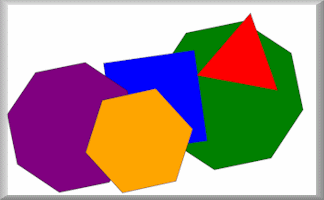#### Mathterpieces

Memorise eight pictures made up of geometrical shapes then sort them into order.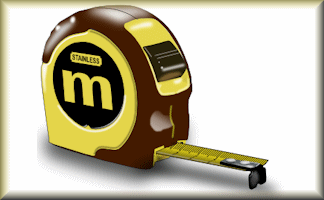#### Memorable Measures

This is a visual aid and printable cards to introduce a homework activity about measures.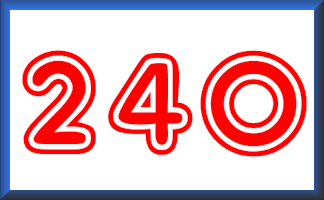#### Pairs 240

Find the pairs of numbers that multiply together to give a product of 240 in this collection of matching games.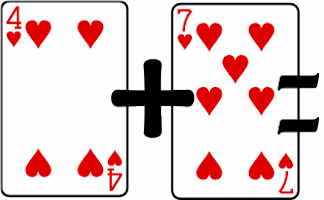#### Pairs Eleven

Find pairs of playing cards of the same suit which add up to eleven.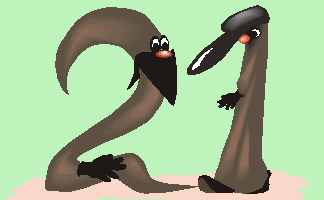#### Pairs Twenty One

Find the pairs of numbers that add up to 21 in this collection of matching games.#### Rotational Symmetry Pairs

The traditional pairs or pelmanism game adapted to test knowledge of rotational symmetry.#### Screen Test

Memorise the mathematical facts in the video then answer the ten quiz questions.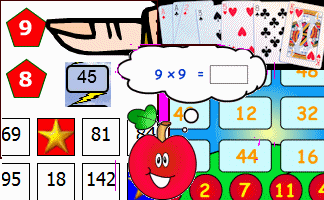#### Times Tables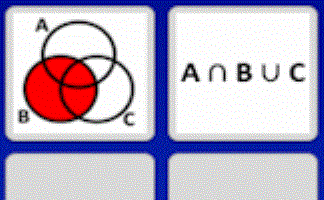#### Venn Diagram Pairs

The traditional pairs or pelmanism game adapted to test knowledge of Venn diagrams.

### Search

The activity you are looking for may have been classified in a different way from the way you were expecting. You can search the whole of Transum Maths by using the box below.

Have today's Starter of the Day as your default homepage. Copy the URL below then select
Tools > Internet Options (Internet Explorer) then paste the URL into the homepage field.

Set as your homepage (if you are using Internet Explorer)

Do you have any comments? It is always useful to receive feedback and helps make this free resource even more useful for those learning Mathematics anywhere in the world. Click here to enter your comments.For All: